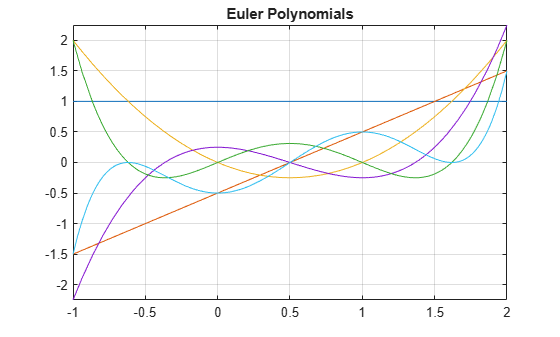# euler

Euler numbers and polynomials

## Syntax

``euler(n)``
``euler(n,x)``

## Description

example

``euler(n)` returns the `n`th Euler number.`

example

````euler(n,x)` returns the `n`th Euler polynomial.```

## Examples

### Euler Numbers with Odd and Even Indices

The Euler numbers with even indices alternate the signs. Any Euler number with an odd index is `0`.

Compute the even-indexed Euler numbers with the indices from `0` to `10`:

`euler(0:2:10)`
```ans = 1 -1 5 -61... 1385 -50521```

Compute the odd-indexed Euler numbers with the indices from `1` to `11`:

`euler(1:2:11)`
```ans = 0 0 0 0 0 0```

### Euler Polynomials

For the Euler polynomials, use `euler` with two input arguments.

Compute the first, second, and third Euler polynomials in variables `x`, `y`, and `z`, respectively:

```syms x y z euler(1, x) euler(2, y) euler(3, z)```
```ans = x - 1/2 ans = y^2 - y ans = z^3 - (3*z^2)/2 + 1/4```

If the second argument is a number, `euler` evaluates the polynomial at that number. Here, the result is a floating-point number because the input arguments are not symbolic numbers:

`euler(2, 1/3)`
```ans = -0.2222```

To get the exact symbolic result, convert at least one number to a symbolic object:

`euler(2, sym(1/3))`
```ans = -2/9```

### Plot Euler Polynomials

Plot the first six Euler polynomials.

```syms x fplot(euler(0:5, x), [-1 2]) title('Euler Polynomials') grid on```### Handle Expressions Containing Euler Polynomials

Many functions, such as `diff` and `expand`, can handle expressions containing `euler`.

Find the first and second derivatives of the Euler polynomial:

```syms n x diff(euler(n,x^2), x)```
```ans = 2*n*x*euler(n - 1, x^2)```
`diff(euler(n,x^2), x, x)`
```ans = 2*n*euler(n - 1, x^2) + 4*n*x^2*euler(n - 2, x^2)*(n - 1)```

Expand these expressions containing the Euler polynomials:

`expand(euler(n, 2 - x))`
```ans = 2*(1 - x)^n - (-1)^n*euler(n, x)```
`expand(euler(n, 2*x))`
```ans = (2*2^n*bernoulli(n + 1, x + 1/2))/(n + 1) -... (2*2^n*bernoulli(n + 1, x))/(n + 1)```

## Input Arguments

collapse all

Index of the Euler number or polynomial, specified as a nonnegative integer, symbolic nonnegative integer, variable, expression, function, vector, or matrix. If `n` is a vector or matrix, `euler` returns Euler numbers or polynomials for each element of `n`. If one input argument is a scalar and the other one is a vector or a matrix, `euler(n,x)` expands the scalar into a vector or matrix of the same size as the other argument with all elements equal to that scalar.

Polynomial variable, specified as a symbolic variable, expression, function, vector, or matrix. If `x` is a vector or matrix, `euler` returns Euler numbers or polynomials for each element of `x`. When you use the `euler` function to find Euler polynomials, at least one argument must be a scalar or both arguments must be vectors or matrices of the same size. If one input argument is a scalar and the other one is a vector or a matrix, `euler(n,x)` expands the scalar into a vector or matrix of the same size as the other argument with all elements equal to that scalar.

collapse all

### Euler Polynomials

The Euler polynomials are defined as follows:

`$\frac{2{e}^{xt}}{{e}^{t}+1}=\sum _{n=0}^{\infty }\mathrm{euler}\left(n,x\right)\frac{{t}^{n}}{n!}\text{\hspace{0.17em}}$`

### Euler Numbers

The Euler numbers are defined in terms of Euler polynomials as follows:

`$\mathrm{euler}\left(n\right)={2}^{n}\mathrm{euler}\left(n,\frac{1}{2}\right)$`

## Tips

• For the other meaning of Euler’s number, e = 2.71828…, call `exp(1)` to return the double-precision representation. For the exact representation of Euler’s number e, call `exp(sym(1))`.

• For the Euler-Mascheroni constant, see `eulergamma`.